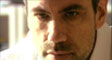David Orrell. Writer and Mathematician

Truth or Beauty: errata

Pg 18. When discussing the Pythagorean ratios of musical consonances, I wrote that the ratios 2:1, 3:2 and 4:3, played on separate strings, would produce a major triad. As McGill music professor Jon Wild pointed out by email, this is incorrect, as "you need a major third, whose ratio is 5:4, for a major triad." He goes on: "The proportion 2:3:4 gives an octave divided 'authentically', in music-historical terms, i.e. at the 5th (C-G-C), if we are talking about frequencies, and an octave divided 'plagally', i.e. at the fourth (C-F-C), if we are talking about string lengths. String lengths were the only known basis for ratios of musical intervals until the late Renaissance. The major triad would be given by the proportion 4:5:6 in terms of frequency, and 10:12:15 in terms of string lengths."

Pg. 51. The speed of light is calculated by dividing the distance traveled by the time, not vice versa as stated.